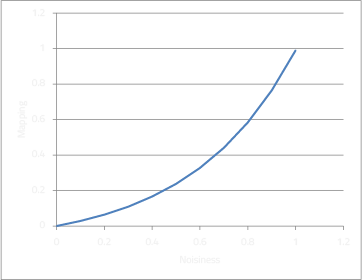## Overview

I developed a sound reactive system to go with a Chinese 5m RGB light strip, which easily lights up the whole room in any colour I want.

It detects the noisiness of the room, and changes colour depending on how noisy it is. When the room is quiet, the room remains a deep blue colour, but it progresses to yellow and red as the room gets noisier.

The system consists of an Arduino and a Python script running on my computer, which listens to an audio input and performs some processing on it to determine the loudness of the room.

## Electronics

The electronics are relatively simple, as all they need to do is receive commands from the computer and drive the LEDs## Arduino

The arduino code is really simple, it just reads 3 binary values from the computer over a serial port, and updates the RGB PWM outputs. I'm using a very simple protocol consisting of 4 bytes, using the first byte to sync the packet in case it gets out of sync.

```// Update LED brightness
void loop()  {
if (Serial.available()) {
if (Serial.read() == 'X') { // sync byte

while (!Serial.available());

while (!Serial.available());

while (!Serial.available());
}
}
}
```

## PythonThe python code does all the heavy audio DSP, and contains the algorithm for determining the colour of the LEDs.

This process is explained below...

### Capturing Audio

To capture audio using python, I'm using pyAudio. It makes it very easy to connect to an audio device and stream data.

To open an audio stream using pyAudio:

```import pyaudio

SAMPLE_RATE = 44100
BUFFER_SIZE = 2**11

p = pyaudio.PyAudio()
stream = p.open(format=pyaudio.paInt16,channels=1,rate=SAMPLE_RATE,\
input=True,output=False,frames_per_buffer=BUFFER_SIZE)
```

Where `BUFFER_SIZE` will determine how long the sample is, and also the frequncy bands generated from the FFT. Thus, changing it will also change the response of the DSP algorithm below, and will need to be adjusted.

### DSP

First we read an audio sample, and convert it to something python can use

```import struct

# Get audio sample
data = scipy.array(struct.unpack("%dh"%(BUFFER_SIZE),buf)) #h: unsigned short, 2 bytes per sample
```

Then we generate an FFT using scipy's FFT library. I'm only interested in the amplitudes, so calling `abs()` on the samples will produce an array of amplitudes.

```from scipy.fftpack import fft, fftfreq
import scipy

def get_fft(data):
""" Run the sample through a FFT """
FFT = fft(data)                                # Returns an array of complex numbers
freqs = fftfreq(BUFFER_SIZE, 1.0/SAMPLE_RATE)  # Returns an array containing the frequency values

y = abs(FFT[0:len(FFT)/2])/1000                # Get amplitude and scale
y = scipy.log(y) - 2                           # Subtract noise floor (empirically determined)
return (freqs,y)

freqs,y = get_fft(fdata)
```

Note that only half of the resulting array has unique data, as the other half is a mirror.

The FFT is then averaged into smaller chunks, which makes it easier to display and process.

```# Average into chunks of N
N = 200
yy = [scipy.average(y[n:n+N]) for n in range(0, len(y), N)]
yy = yy[50:]  # Discard half of the samples, as they are mirrored
```

Sidenote: You could probably skip using FFT and just take the absolute average of the signal, depending on how you want the system to react.

### RGB Algorithm

The loudness level is determined using a threshold detection system

```def thresh(val, threshold):
val -= threshold
if val < 0: val = 0
val *= (1.0/threshold)
return val

CHANNEL = 1      # which FFT frequency to respond to
GAIN = 1.5       # audio gain
THRESHOLD = 0.15 # audio trigger threshold

loudness = thresh(yy[CHANNEL] * GAIN, THRESHOLD)
```

The loudness level is based on an attack-decay system, in that it will decay over quiet periods, and build up over louder periods.

```ATTACK = 0.004  # amount of increase with loudness
DECAY = 0.003   # amount of decay

noisiness -= DECAY
noisiness += loudness * ATTACK
noisiness = limit(noisiness, 0.0, 1.0)
```

The noisiness value is now the mapping that will decide which hue to use. I wanted it to spend less time at the red end, and more time in the green/yellow end, so I applied a power function to it:

```mapping = (10 ** limit(noisiness, 0.0, 1.0)) / 10.0                 # Power function
mapping = mapping * 1.1 - 0.11                                      # Linear correction
```Finally this is turned into a hue value

```MIN_HUE = 200  # Aqua
MAX_HUE = 0    # Red
hue = mapval(limit(noisiness, 0.0, 1.0), 0.0, 1.0, MIN_HUE, MAX_HUE)
```

Where `mapval()` is a linear mapping between values, and `limit()` truncates values outside the specified values. See the source code for the actual implementation of it.

Now we can update the LEDs

```red,green,blue = hsv2rgb(hue,1.0,brightness)
rgb_update([int(red),int(green),int(blue)])
```

I obtained `hsv2rgb` from here: Python RGB and HSV Conversion

### Communication

I'm using a very simple protocol, and `pyserial` to communicate with the arduino.

```import struct
import serial

ser = serial.Serial('COM3') # Change as appropriate

def tobyte(i):
i = int(i)
if i < 0: i = 0
if i > 255: i = 255
return i

def rgb_update(color):
r = tobyte(color)
g = tobyte(color)
b = tobyte(color)
packet = struct.pack('cBBB', 'X', r,g,b)
#print packet
self.ser.write(packet)
```